# How to get month from week number and year in Excel?

This article briefs learners about the way to generate the month-from-week number and year in Excel. This article describes two strategies to achieve the required task. The first example guides the learner to use the predefined methods, in the form of user-defined formulas. The second method guides the learner about the use of VBA code. Please note that VBA is a programming language that can work only when written properly within a proper format. This article contains stepwise explanations for both the discussed practices.

## Example 1: To get month from week number and year in Excel by using the formula:

### Step 1:

To understand the process of generating month numbers from the provided week number and year. Consider the below-provided worksheet, with some sample data −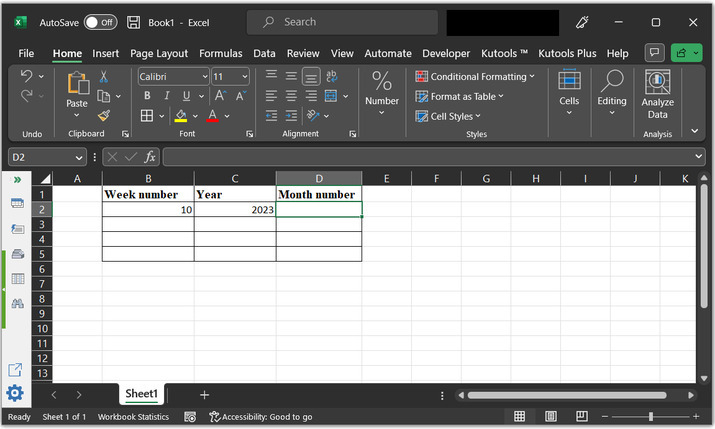### Step 2:

Go to the D2 cell and type the mentioned formula to obtain the required month “=MONTH(DATE(C2,1,B2,*7-2)-WEEKDAY(DATE(B2, 1, 3)))” as highlighted in the below image.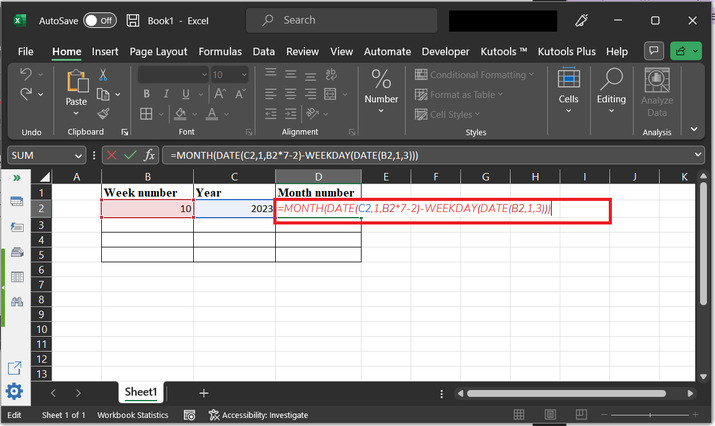### An explanation for the formula:

• DATE(C2,1, B2,*7-2) − this part of the formula creates a date for the first day of the specified year (referenced by using the C2 cell). Here, the month will be set to January.

• WEEKDAY(DATE(B2, 1, 3)) − this part of the formula calculates the day of the week for January 3 of the specified year ( referenced in cell C2). It is compulsory because Excel's week numbering system considers the week containing January 1 as the first week of the year.

• DATE(C2,1, B2,*7-2)-WEEKDAY(DATE(B2, 1, 3)) − this part will subtract the day of the week for January 3 of the specified year from the date calculated in step 1. This provides the date for the first day of the first week of the specified year.

• Finally MONTH() method will calculate the month for the date calculated in the above step. This is the month that contains the specified week number (in cell B2) of the specified year (in cell C2).

### Step 3:

Press the “Enter” key. This will display the result in the current row and will shift the control to the next box. Consider the below-given image for reference.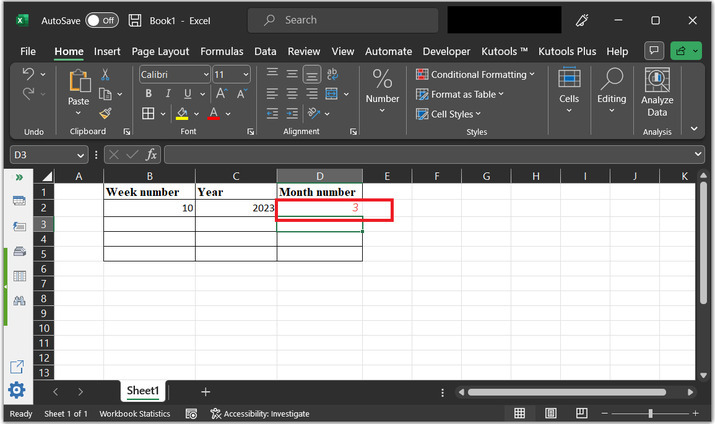## Example 2: To get month from week number and year in Excel by using the VBA code:

### Step 1:

To understand the process of using the VBA code, to evaluate month numbers from weekend and year, consider an empty worksheet.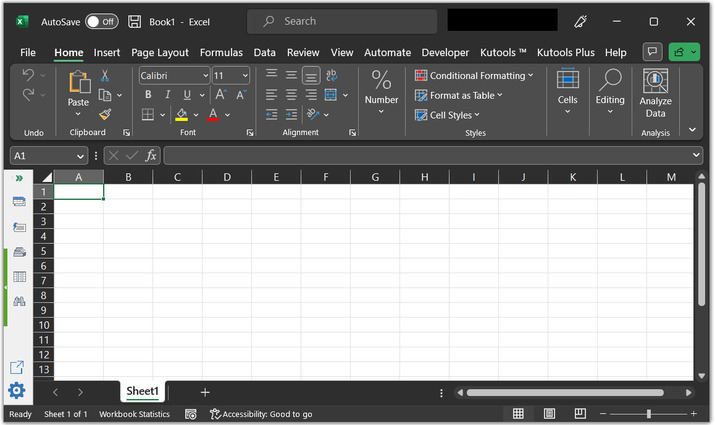### Step 2:

To open the code editor, go to the “Developer”→ Visual Basic, under the “code” section, as shown in the below image.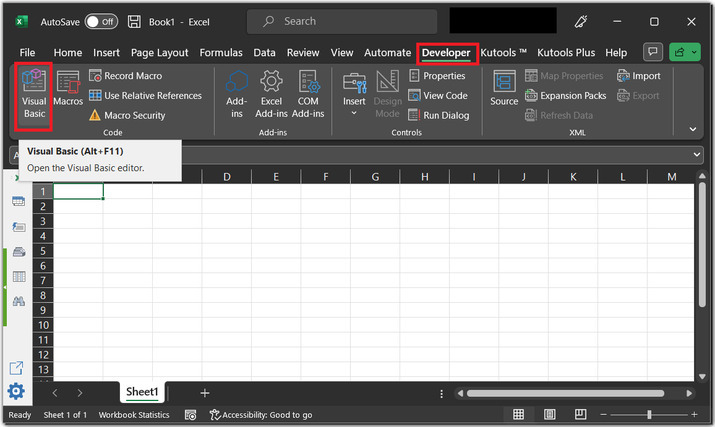### Step 3:

The above step will open a “Microsoft Visual Basic for Applications” dialog as depicted below: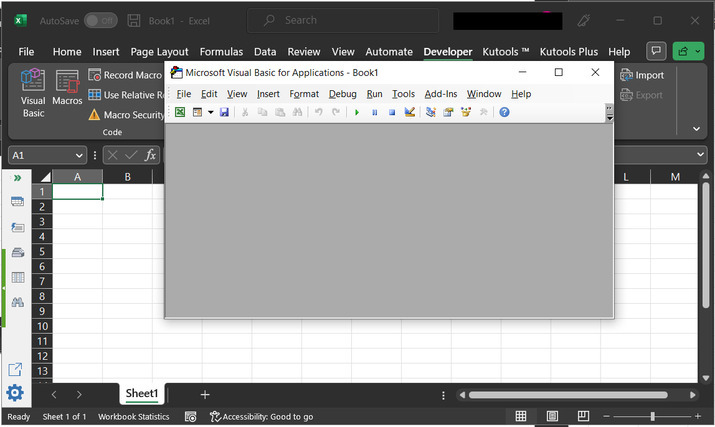### Step 4:

In the opened dialog box, select “Insert”, and then click on the “Module” option as highlighted in the below image: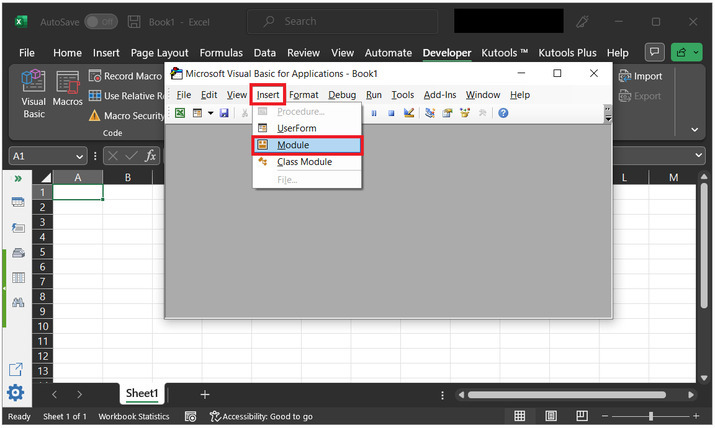### Step 5:

This will open the code area, as highlighted below: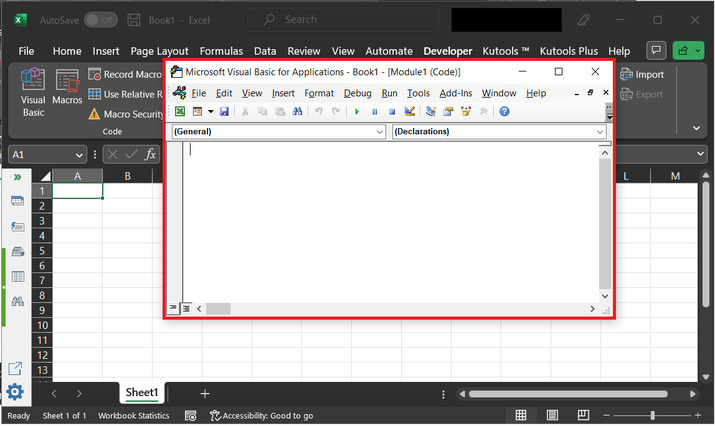### Step 6:

Copy the below given code, to the code area:

'define function to get month from provide weeknumber Function GetMonthFromWeekNumber(weekNum As Integer, yearNum As Integer) As Integer

    ' declaring required variables
Dim firstDayOfYear As Date
' storing data in above declared method
firstDayOfYear = DateSerial(yearNum, 1, 1)
' declare the variable to store first week starting date
Dim firstWeekStart As Date
' calculate the date and store it in the above variable
firstWeekStart = DateAdd("d", 1 - Weekday(firstDayOfYear, vbMonday), firstDayOfYear)
' declare variable
Dim targetWeekStart As Date
' store data to above declared variable
targetWeekStart = DateAdd("ww", weekNum - 1, firstWeekStart)
' calling method name as a constructor
GetMonthFromWeekNumber = Month(targetWeekStart)
' end of the function definition
End Function


### Consider the snapshot of code area: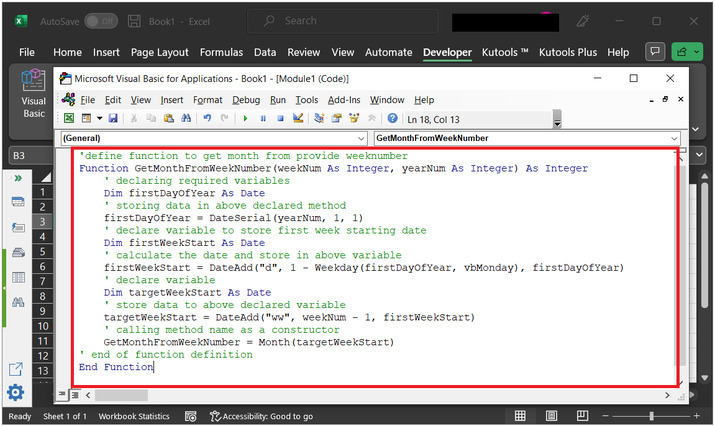### Step 7:

Again go to the Excel sheet, and type ‘=GetMonthFromWeekNumber(10,2023)’. The specified command will accept the week number and year number as an argument.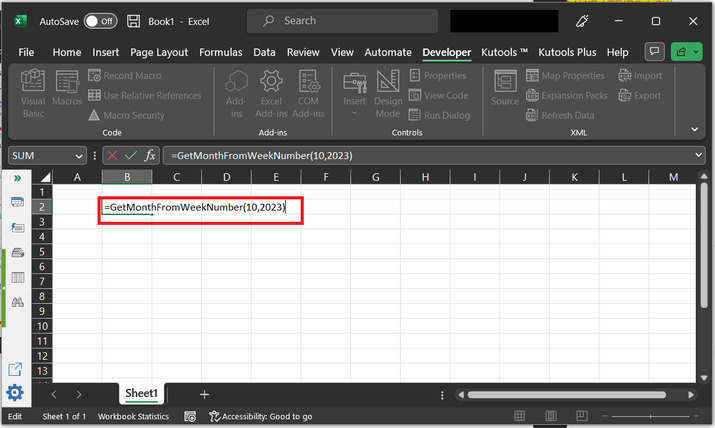### Step 8:

Press the “Enter” key. This will allow the user to generate the output, as shown below: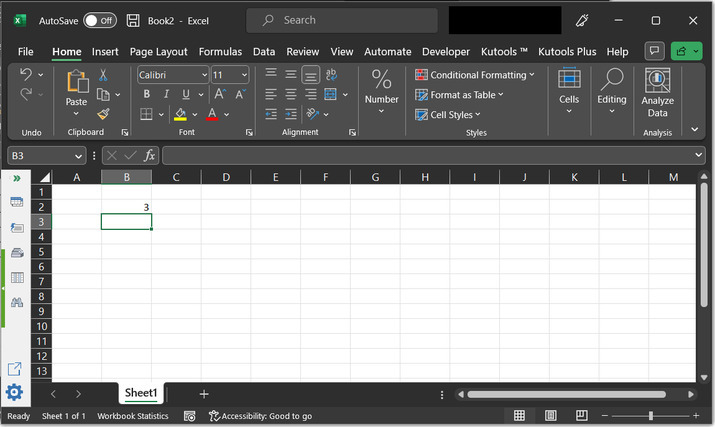## Conclusion:

In this article, the user will easily obtain the month number by using the weekday and year numbers. This article describes two examples to perform the same tasks, the first example is based on using the predefined formula, and the second is based on the VBA coding language. The step-by-step description along with screenshots are explained in both examples.

Updated on: 17-Apr-2023

7K+ Views